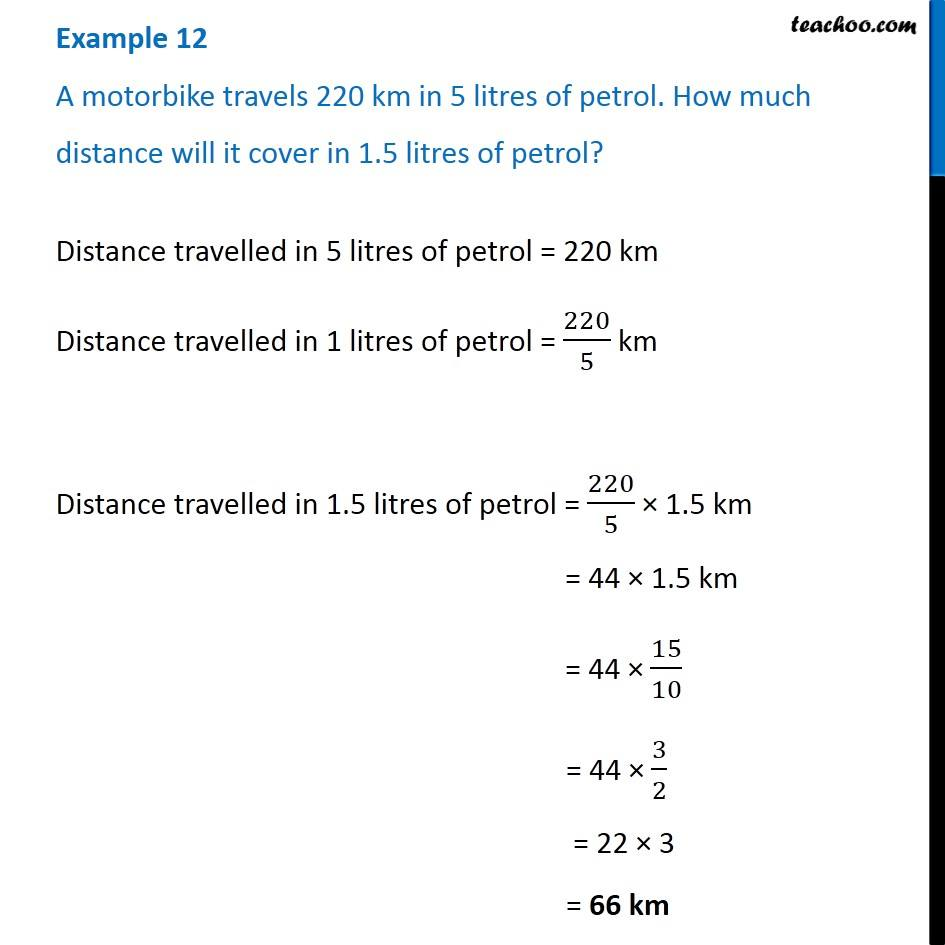Examples

Chapter 12 Class 6 Ratio And Proportion
Serial order wiseGet live Maths 1-on-1 Classs - Class 6 to 12

### Transcript

Example 12 A motorbike travels 220 km in 5 litres of petrol. How much distance will it cover in 1.5 litres of petrol?Distance travelled in 5 litres of petrol = 220 km Distance travelled in 1 litres of petrol = 220/5 km Distance travelled in 1.5 litres of petrol = 220/5 × 1.5 km = 44 × 1.5 km = 44 × 15/10 = 44 × 3/2 = 22 × 3 = 66 km